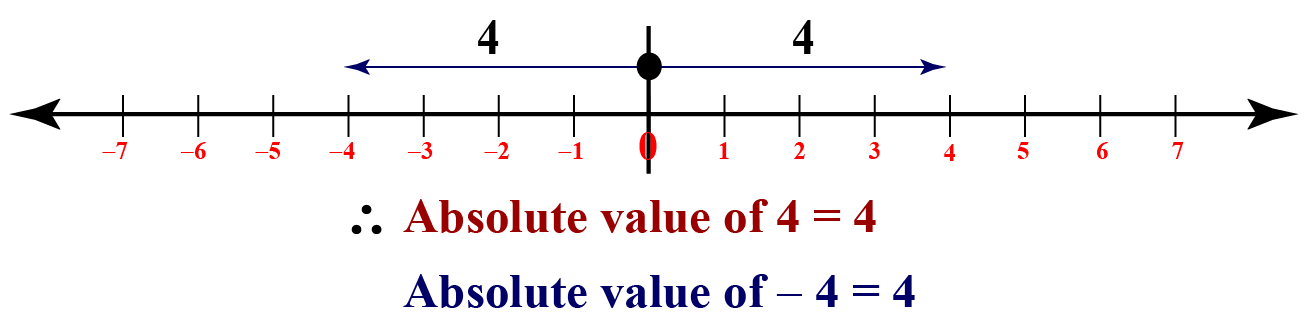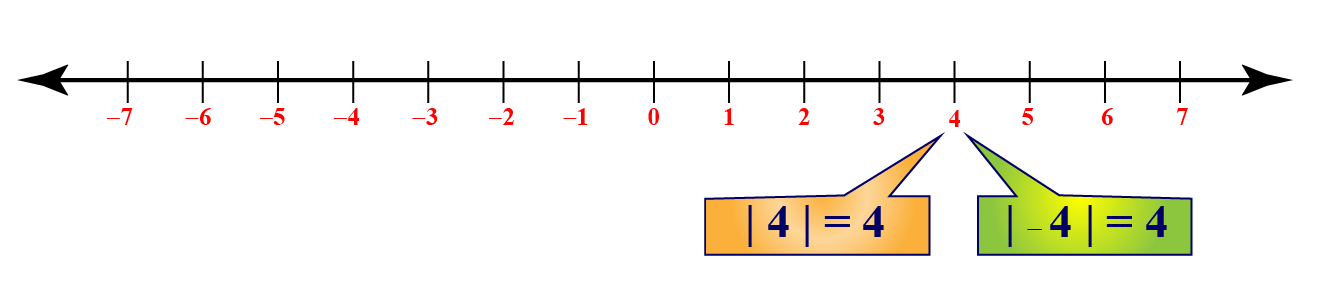# Absolute Value

In this fun lesson, we will explore the world of Absolute Value. The journey will take us through the absolute value symbol, absolute value function, absolute value examples, and absolute value calculator. You can check out the interactive calculator to know more about the lesson and try your hand at solving a few interesting practice questions at the end of the page.

The absolute value of a variable $$x$$ is represented by $$|x|$$ which is pronounced as 'Mod x' or 'Modulus of x'.

• 'Modulus' is a Latin word, which means 'measure'.
• Other names of 'Absolute Value' are 'Numerical value' and 'Magnitude'.

We can observe the absolute values on the number line using the following illustration.## Lesson Plan

 1 What Are Absolute Values? 2 Important Notes on Absolute Value 3 Thinking out of the Box! 4 Solved Examples on Absolute Value 5 Interactive Questions on Absolute Value

## What Are Absolute Values?

The absolute value of a number is its distance from 0.

We know that distance is a non-negative quantity always.

Since the absolute value is a distance, the absolute value is always non-negative.

## What Is the Absolute Value Symbol?

To represent the absolute value of a number (or a variable), we write a vertical bar on either side of the number.

For example:

• The absolute value of 4 is written as |4|
• The absolute value of -4 is written as |-4|

As we discussed earlier, the absolute value results in a non-negative value all the time. Hence,

$|4|=|-4| =4$

That is, it turns negative numbers also into positive numbers.

Thus, the above figure with the absolute value symbols is:## Absolute Value Calculator

Here is the Absolute Value Calculator.

This allows you to enter any number and calculates the absolute value of the number you have entered.

## Solved Examples

Here are some absolute value examples.

 Example 1

Mohan wants to find the values of the following. Shall we help her using the definition of absolute value?

a) $$\left|\dfrac{-13}{15}\right|$$

b) $$- |-3|$$

c) $$|2(-3) +4|$$

Solution

We know that absolute value results in non-negative values all the time.

Thus,

\begin{align} a) \,\, \left|\dfrac{-13}{15}\right| &= \dfrac{13}{15}\\[0.2cm]b)\,\, - |-3| &= -3 \,\, [\because |-3|=3]\\[0.2cm] c)\,\,|2(-3) +4| &= |-6+4| = |-2|=2\end{align}

 \begin{align}\therefore\ &a) \,\, \left|\dfrac{-13}{15}\right| = \dfrac{13}{15}\\[0.2cm] & b)\,\, - |-3| = -3\\[0.2cm]&c) \,\,|2(-3) +4|=2 \end{align}
 Example 2

Ria is instructed by her teacher to solve the following absolute value equation using the definition of the absolute function.

$|x-2|=4$

Can we try to help her?

Solution

The given equation is:

$|x-2|=4$

Using the definition of absolute value function, we remove the absolute value sign on one side of the equation. We then get $$\pm$$ sign on the other side.

$x-2=\pm 4$

This results in two equations, which we solve separately.

\begin{align} x-2&=4 & x-2&=-4\\[0.2cm] x &= 6 & x &=-2 \end{align} Thus, the solutions of the given equation are:

 \begin{align}\therefore\ x&=6\\[0.1cm] x&=-2 \end{align}
 Example 3

Rohan wants to find the value of $$-|-x|$$ when $$x>0$$.

Let's help him using the definition of the absolute value function.

Solution

It is given that $$x>0$$.

Then definitely, $$-x<0$$.

By the definition of the absolute value function,

$|-x|= -(-x) = x$

 $\therefore\ -|-x|= -x$

## Interactive Questions

Here are a few activities for you to practice. Select/Type your answer and click the 'Check Answer' button to see the result.

## Let's Summarize

We hope you enjoyed learning about Absolute Value with the simulations and practice questions. Now you will be able to easily solve problems on absolute value symbol, absolute value function, absolute value examples, and absolute value calculator.

At Cuemath, our team of math experts is dedicated to making learning fun for our favourite readers, the students!

Through an interactive and engaging learning-teaching-learning approach, the teachers explore all angles of a topic.

Be it worksheets, online classes, doubt sessions, or any other form of relation, it’s the logical thinking and smart learning approach that we at Cuemath believe in.

## 1. What is the absolute value of 10?

The absolute value of 10 is 10 itself, i.e., $|10|=10$

## 2. What is the absolute value of |-11|?

The absolute value of |-11| is 11 because absolute value turns negative numbers into positive numbers, i.e., $|-11|=11$

## 3. What is the absolute value of 8?

The absolute value of 8 is 8 itself, i.e., $|8|=8$

More Important Topics
Numbers
Algebra
Geometry
Measurement
Money
Data
Trigonometry
Calculus